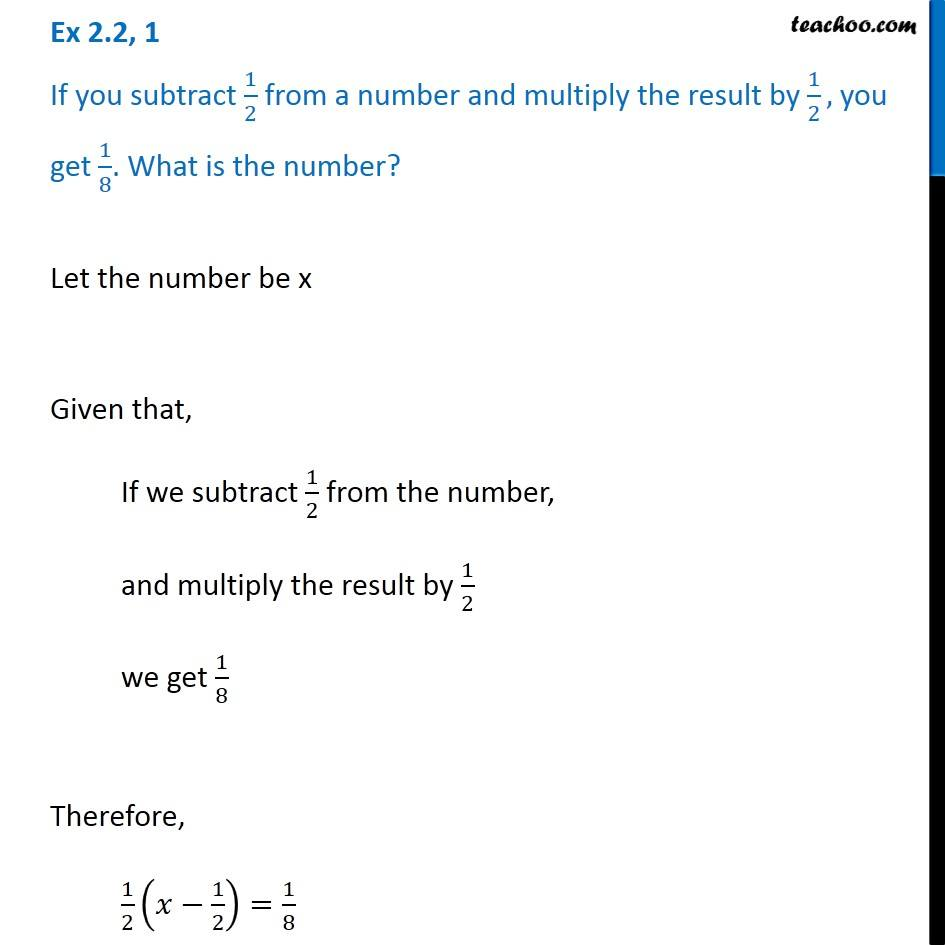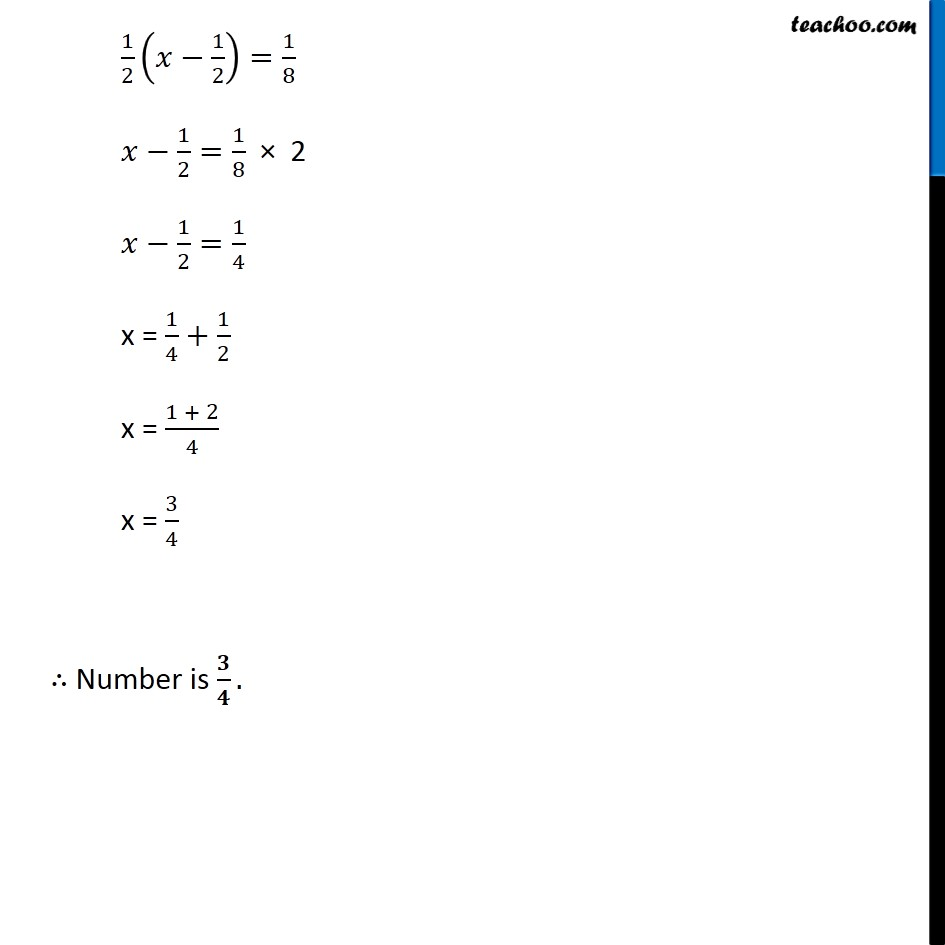Solving easy equations - Word Problems

Chapter 2 Class 8 Linear Equations in One Variable
Serial order wiseLearn in your speed, with individual attention - Teachoo Maths 1-on-1 Class

### Transcript

Question 1 If you subtract 1/2 from a number and multiply the result by 1/2, you get 1/8. What is the number? Let the number be x Given that, If we subtract 1/2 from the number, and multiply the result by 1/2 we get 1/8 Therefore, 1/2 (𝑥 − 1/2) = 1/8 1/2 (𝑥 − 1/2) = 1/8 𝑥 − 1/2 = 1/8 × 2 𝑥 − 1/2 = 1/4 x = 1/4 + 1/2 x = (1 + 2)/4 x = 3/4 ∴ Number is 3/4